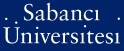# Multicommodity network flow problem with substitution

Köker, Ekin (2013) Multicommodity network flow problem with substitution. [Thesis]Preview
PDF - Requires a PDF viewer such as GSview, Xpdf or Adobe Acrobat Reader
888Kb

## Abstract

Multicommodity network flow problems are generalizations of single commodity network flow problems, where a number of commodities flow through the network often sharing common resources such as arc capacities. While the single commodity problem can be solved in polynomial time even when the flow quantities are imposed as integer values only, the integer multicommodity version of the problem with arc capacities is NPhard. We introduce a generalization of the multicommodity network flow problem where substitution is possible amongst commodities. We develop mathematical models as the linear integer programming formulations of two-commodity and three-commodity problems with both commodity-specific and overall arc capacities. We prove that constraint matrices are totally unimodular in the mathematical programming formulations for the uncapacitated versions. We investigate the empirical computational difficulty of capacitated versions of the problem formulations through a computational study with randomly generated problems and statistical analysis with hypothesis testing. In particular, we explore the effect of capacities and the problem size on solution time. Our results show that solution time significantly increases for both two-commodity and three-commodity problems when both overall and commodity-specific capacities exist. Solution time significantly increases when problem size is increased. Finally, we generalized the two and three-commodity models for the multicommodity problem.

Item Type: Thesis Multicommodity network flows. -- Substitutable products. -- Unimodular matrices. -- NP-hardness. -- Çok ürünlü ağ akışı. -- Akış problemi. -- İkame edilebilir ürünler. -- Ünimodüler matrisler. -- NP-zorluk. T Technology > T Technology (General) > T055.4-60.8 Industrial engineering. Management engineering 31015 IC-Cataloging 30 Dec 2016 12:19 22 May 2019 13:45

Repository Staff Only: item control page